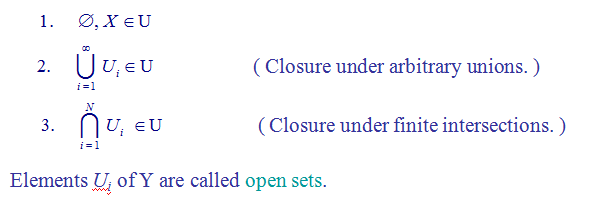# SCC Education

## Geometrical Methods of Mathematical Physics

Geometrical Methods of Mathematical Physics

1.Some Basic Mathematics
2.Differentiable Manifolds And Tensors
3.Lie Derivatives And Lie Groups
4.Differential Forms
5.Applications In Physics

6.Connections For Riemannian Manifolds And Gauge Theories

Basic Algebraic Structures

Structures with only internal operations:
Group ( G, × )
Ring ( R, +, × ) : ( no e, or x-1 )
Field ( F, +, × ) : Ring with e & x-1 except for 0.
Structures with external scalar multiplication:
Module ( M, +,  ×  ;  R )
Algebra ( A, +,  ×  ;  R with e )
Vector space ( V, + ; F )

Prototypes:
R is a field.
Rn is a vector space
The Space Rn And Its Topology
Goal:  Extend multi-variable calculus (on En) to curved
spaces without metric.

Bonus:  vector calculus on E3 in curvilinear
coordinates
Basic calculus concepts & tools (metric built-in):

Limit, continuity, differentiability, …

r-ball neighborhood,  δ-ε formulism, …

Integration, …

Essential concept in the absence of metric:

Proximity → Topology.

A system U of subsets Ui of a set X defines a topology on X ifA topological space is the minimal structure on which concepts of     neighborhood,   continuity,   compactness,   connectedness can be defined.

Trivial topology:    U = { Æ, X  }
→  every function on X is dis-continuous
Discrete topology:   U = 2X
→  every function on X is continuous
Exact choice of topology is usually not very important:
2 topologies are equivalent if there exists an homeomorphism (bi-continuous bijection) between them.
Tools for classification of topologies:
topological invariances,  homology,  homotopy, …